AWS Global Infrastructure

### Programming & Frameworks

Topics Covered
• C Programming and Data Structures (17 Blogs)
• Comprehensive Java Course (4 Blogs)
• Java/J2EE and SOA (339 Blogs)
• Spring Framework (8 Blogs)
SEE MORE

# How To Carry Out Swapping of Two Numbers in C?How To Carry Out Swapping of Two Numbers in C?

Swapping two number in C programming language means exchanging the values of two variables. Suppose you have two variable var1 & var2. Value of var1 is 20 & value of var2 is 40. So, after swapping the value of var1 will become 40 & value of var 2 will become 20. In this blog will understand how to swap two variables in C.

We will look at each one of them one by one.

## Swapping Two Numbers Using Third Variable

Logic
The idea behind swapping two numbers using 3rd variable is simple. Store the value of 1st variable in temporary variable. Store the value of 2nd variable in the first variable. At last, store the value of temp variable in 2nd variable. In this program, we are using a temporary variable to hold the value of the first variable.

• Assign var1 value to a temp variable: temp = var1

• Assign var2 value to var1: var1 = var2

• Assign temp value to var2: var2 = temp

Code:

```temp = var1;
var1 = var2;
var2 = temp;
```

Now, let’s look at the complete code.

Example

```#include <stdio.h>
int main()
{
int var1, var2, temp;
printf("Enter two integersn");
scanf("%d%d", &var1, &var2);
printf("Before SwappingnFirst variable = %dnSecond variable = %dn", var1, var2);
temp = var1;
var1 = var2;
var2 = temp;
printf("After SwappingnFirst variable = %dnSecond variable = %dn", var1, var2);
return 0;
}

```

Output: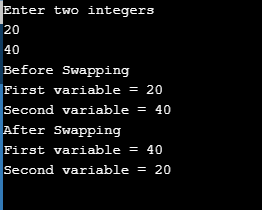Moving on with this article on Swapping of Two Numbers in C

## Swapping Two Numbers Using Without Using Third Variable

In this variation of swapping two variables, we are not using any temporary variable to store the value. In the first variable we are storing the sum of both variable. Then, in next step we are we are extracting the value of 1st variable by subtracting the value of 2nd variable form the sum & storing it in 2nd variable. At last, we are extracting the original value of the 2nd variable & storing it in the 1st variable.

Code:

```var1 = var1 + var2;
var2 = var1 - var2;
var1 = var1 - var2;
```

Now, let’s look at the complete code.

Example

```#include <stdio.h>
int main()
{
int var1, var2, temp;
printf("Enter two integersn");
scanf("%d%d", &var1, &var2);
printf("Before SwappingnFirst variable = %dnSecond variable = %dn", var1, var2);
var1 = var1 + var2;
var2 = var1 - var2;
var1 = var1 - var2;
printf("After SwappingnFirst variable = %dnSecond variable = %dn", var1, var2);
return 0;
}
```

Output: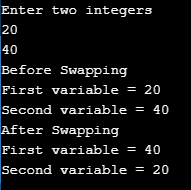Moving on with this article on Swapping of Two Numbers in C

## Swapping Function in C

You can create a swapping function in C implementing any of the swapping variation, wherein you can call the function anytime when you want to swap two variables. Since we want the local variables of main to modified by swap function, we must them using pointers.

Example

```void swap(int *num1, int *num2)
{
int temp = *num1;
*num1 = *num2;
*num2 = temp;
}
int main()
{
int var1, var2;
printf("Enter Value of var1 ");
scanf("%d", &var1);
printf("nEnter Value of var2 ");
scanf("%d", &var2);
swap(&var1, &var2);
printf("nAfter Swapping: var1 = %d, var2 = %d", var1, var2);
return 0;
}
```

Output: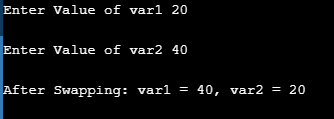Moving to the next variation we have swapping two numbers using pointers.

## Swap two numbers using pointers in C

You can also swap two variables using pointers, wherein you pass the address of the variables to two different variables. Then, swap their values.

Code

```num1 = &var1;
num2 = &var2;
temp = *num2;
*num2   = *num1;
*num1   = temp;
```

Now, let’s look at the complete code.

Example

```#include <stdio.h>
int main()
{
int var1, var2, *num1, *num2, temp;
printf("Enter the value of var1 and var2n");
scanf("%d%d", &var1, &var2);
printf("Before Swappingnvar1 = %dnvar2 = %dn", var1, var2);
num1 = &var1;
num2 = &var2;
temp = *num2;
*num2   = *num1;
*num1   = temp;
printf("After Swappingnvar1 = %dnvar2 = %dn", var1, var2);
return 0;
}
```

Output: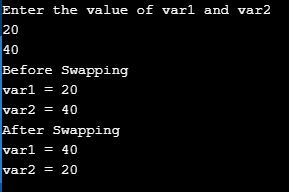Moving to the last variation of swapping variables in C. Let’s understand how to swap two variables using bitwise XOR operator.

## Swap Two Numbers Using Bitwise XOR

XOR operator works in the similar manner as swapping without using temporary variable works. We extract calculate the XOR of both the variables. Then we extract individual values and swap them.

Suppose var1 = 20 and var2 = 40. The binary values of 20 = 10100 and 40 = 101000.

Example:

Var1 = Var1 ^ Var2
Var1 = 10100 ^ 101000
Var1 = 111100

Var2 = Var1 ^ Var2
Var2 = 111100 ^ 101000
Var2 = 10100

Var1 = Var1 ^ Var2;
Var1 = 111100 ^ 10100
Var1 = 101000
Final Values after swapping 2 numbers: var1 = 40 and var2 = 20

var1 = var1 ^ var2;
var2 = var1 ^ var2;
var1 = var1 ^ var2;

Now, let’s look at the complete code.

```#include <stdio.h>
int main()
{
int var1, var2, temp;
printf("Enter two integersn");
scanf("%d%d", &var1, &var2);
printf("Before SwappingnFirst variable = %dnSecond variable = %dn", var1, var2);
var1 = var1 ^ var2;
var2 = var1 ^ var2;
var1 = var1 ^ var2;
printf("After SwappingnFirst variable = %dnSecond variable = %dn", var1, var2);
return 0;
}
```

Output: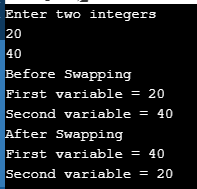Now after going through the above programs you would have understood all the variations of swapping two numbers in C. I hope this blog is informative and added value to you.

Now after executing the above program you would have understood the Swapping of Two Numbers in C. Thus we have come to an end of this article on ‘Quicksort in Java’. If you wish to learn more, check out the Java Training by Edureka, a trusted online learning company. Edureka’s Java J2EE and SOA training and certification course is designed to train you for both core and advanced Java concepts along with various Java frameworks like Hibernate & Spring.

Got a question for us? Please mention it in the comments section of this blog and we will get back to you as soon as possible.

Upcoming Batches For Java Certification Training Course
Course NameDate
Java Certification Training Course

Class Starts on 28th May,2022

28th May

SAT&SUN (Weekend Batch)
View Details
Java Certification Training Course

Class Starts on 11th June,2022

11th June

SAT&SUN (Weekend Batch)
View Details
Java Certification Training Course

Class Starts on 25th June,2022

25th June

SAT&SUN (Weekend Batch)
View DetailsREGISTER FOR FREE WEBINAR
Send OTPThank you for registering Join Edureka Meetup community for 100+ Free Webinars each month

### Subscribe to our Newsletter, and get personalized recommendations.#### How To Carry Out Swapping of Two Numbers in C?

edureka.co